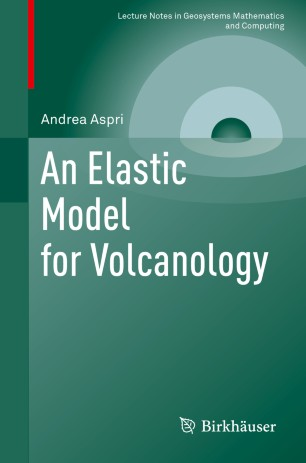# An Elastic Model for Volcanology

• Andrea AspriBook

1. Front Matter
Pages i-x
2. Andrea Aspri
Pages 1-10
3. Andrea Aspri
Pages 11-51
4. Andrea Aspri
Pages 53-118
5. Back Matter
Pages 119-126

### Introduction

This monograph presents a rigorous mathematical framework for a linear elastic model arising from volcanology that explains deformation effects generated by inflating or deflating magma chambers in the Earth’s interior. From a mathematical perspective, these modeling assumptions manifest as a boundary value problem that has long been known by researchers in volcanology, but has not, until now, been given a thorough mathematical treatment. This mathematical study gives an explicit formula for the solution of the boundary value problem which generalizes the few well-known, explicit solutions found in geophysics literature. Using two distinct analytical approaches—one involving weighted Sobolev spaces, and the other using single and double layer potentials—the well-posedness of the elastic model is proven. An Elastic Model for Volcanology will be of particular interest to mathematicians researching inverse problems, as well as geophysicists studying volcanology.

### Keywords

Mathematical geophysics Mathematical geosciences Mathematical geophysics book Mathematical modeling geophysics Mogi model linear elasticity magma chamber hydrostatic pressure Mogi model Neumann boundary problem Half-space model Magma chamber Geophysics research math Math research volcanoes Math magma asymptotic expansions single and double layer potentials neumann function stability estimates weighted sobolev spaces

• Andrea Aspri
• 1